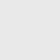## What is the Binary Number system?

The binary number system is used to define numbers in a binary number system. The binary system is used to represent numbers with only two digits, 0 and 1. The binary number system is commonly used in computer languages ​​such as Java and C++. Computers only understand a binary language of 0s or 1s, so any input given to a computer is decoded into a series of 0s or 1s for further processing.

This is a positioning system. Therefore, each binary digit is a power of 2 starting at 20 on the right. In a binary number system, each binary digit represents one bit.   For example, the binary number 1011 represents:
1 x 2+ 0 x 22 + 1 x 21 + 1 x 2
= 8 + 0 + 2 + 1
= 11

## What is the Hexadecimal system (Hex)?

Hex is used in mathematics and information technology as a more convenient way to represent binary numbers. Each hexadecimal number represents four binary digits. So Hex is a language for writing binary files in short form.

The digits 0-9 represent the values ​​0-9 and the letters A-F represent the hexadecimal values ​​10-15. Each digit position represents a power of 16. It starts at 160 (1) and increases by a factor of 16 for each position to the left. For example, the hexadecimal number 3A7B is represented as:

(3A7B)16 = (0011101001111011)2

## How to Convert Binary to Hex?

To convert binary to hexadecimal numbers, we need to use both the base numbers i.e. 2 for binary and 16 for hexadecimal. There are various confusing methods of conversion but for you, we picked the most easy and quick which is using the binary to hexadecimal conversion table where 1 hexadecimal number is equivalent to 4 binary numbers.

Let us see the Binary to a hexadecimal method in detail.

In the grouping approach, there are only 16 digits (from 0 to 7 and A to F) in the hexadecimal number system, so we can represent any digit of the hexadecimal number system using only 4 bits. Follow the steps below:

• Write down the binary number and group the digits (0’s and 1’s) in sets of four. Start doing this from the right. If the leftmost group doesn’t have enough digits to make up a set of four, add extra 0’s to make a group.
• Write 8, 4, 2, and 1 below each group. These are the weights of the positions or placeholders in the numbers (23, 22, 21, and 20).
• Every group of four in binary will give you one digit in hexadecimal. Multiply the 8, 4, 2, and 1’s by the digit above.
• Add the products within each set of four. Write the sums below the groups they belong to.
• The digits you get from the sums in each group will give you the hexadecimal number, from left to right.

Let us understand this process with the help of an example. Here is an example to illustrate the process:

Ex: convert the binary number 110110111011 to hexadecimal.

1. Write down the binary number: 110110111011
2. Group the binary digits into sets of 4: (1101) (1011) (1011)
3. Write down the hexadecimal equivalent of each group of 4 binary digits:
1. 1101 = D1011 = B
1. 1011 = B

## Identify HEX

There are various other prefixes and suffixes specific to particular programming languages. For example, assembly language may use the ‘H’ or ‘h’ suffix (e.g. 7Fh) or the ‘$’ prefix ($6AD). If you’re not sure which prefix or suffix to use for your programming language, check out the examples.

## Subscribe to our weekly newsletter

Subscribe
Notify ofInline Feedbacks

## Browse## Browse